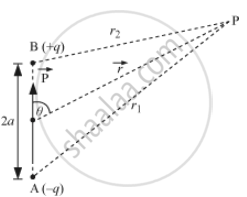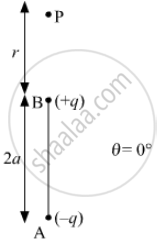PUC Karnataka Science Class 12Department of Pre-University Education, Karnataka
Share

# Derive the Expression for the Electric Potential Due to an Electric Dipole at a Point on Its Axial Line. - PUC Karnataka Science Class 12 - Physics

#### Question

Derive the expression for the electric potential due to an electric dipole at a point on its axial line.

#### Solution

i) Let P be the point at which electric potential is required.Potential at P due to − q charge,

V_1=-q/(4piepsilon_0r_1)

Potential at P due to + q charge,

V_2=q/(4piepsilon_0r_2)

Potential at P due to the dipole,

V=V_1+V_2

V=q/(4piepsilon_0)[1/r_2-1/r_1]   .....(i)

Now, by geometry

r_1^2=r^2+a^2+2arcostheta

r_2^2=r^2+a^2+2arcos(180^@-theta)
r_2^2=r^2+a^2-2arcostheta

r_1^2=r^2(1+a^2/r^2+(2a)/rcostheta)

If r >> a, a/r is small. Therefore,a^2/r^2 can be neglected.

r_1^2=r^2(1+(2a)/rcostheta)

1/r_1=1/r(1+(2a)/rcostheta)^(1/2)

similarly, 1/r_2=1/r(1-(2a)/rcostheta)^(1/2)

Putting these values in (i), we obtain

V=q/(4piepsilon_0)[1/r(1-(2a)/rcostheta)^(1/2)-1/r(1+(2a)/rcostheta)^(1/2)]

Using Binomial theorem and retaining terms up to the first order ina/r, we obtain

V=q/(4piepsilon_0r)[1+a/rcostheta-(1-a/rcostheta)]

V=q/(4piepsilon_0r)[1+a/rcostheta-1+a/rcostheta]

V=q/(4piepsilon_0r)[(2a)/rcostheta]

V=(qxx2acostheta)/(4piepsilon_0r^2)

V=(Pcostheta)/(4piepsilon_0r^2)For axial line put θ=0°

V=(Pcos(0))/(4piepsilon_0r^2)

V=P/(4piepsilon_0r^2)

Is there an error in this question or solution?

#### Video TutorialsVIEW ALL 

Solution Derive the Expression for the Electric Potential Due to an Electric Dipole at a Point on Its Axial Line. Concept: Electric Dipole.
S